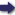(London :  Kegan Paul, Trench, Trübner & Co.,  1910.)

 Tools

## Search this bookPrev Page 245 Next``` CHAPTER XXII I. 24S the point A. This line lies in the plane of the horizon as far as the human eye reaches round the earth. We join the points A and H with each other, and draw the line H B C, so that it is met in C by the line A C. Further, we let fall the perpendicular A T on H C. Now, it is evident that— A T is the sine of the greatest declination ; T B the versed sine of the greatest declination ; T H the sine of the complement of the greatest declination. And as we here occupy ourselves with Aryabhata, we shall, according to his system, change the sines in kardajdt. Accordingly— AT = 1397. TH = 3140. B T = 298. Because the angle H A C is a right angle, we have the equation— HT:TA = TA:TC. And the square of A T is 1,951,609. If we divide it by T H, we get as quotient 622. The difference between this number and T B is 324, which is B C. And the relation of B C to B H, the latter being si^ius totus=T)4.2)^, is the same as the relation of the number of yojanas of B C to the yojanas of B H. The latter number is, according to Aryabhata, 800. If it is multiplied by the just-mentioned difference of 324 we get the sum of 259,200. And if we divide this number by the sinus totus we get 75 as quotient, which is the number of yojanas of B C, equal to 600 miles or 200 farsakh. If the perpendicular of a mountain is 200 farsakh, the ascent will be nearly the double. Whether Mount Meru has such a height or not, nothing of it can be visible in the 66th degree of latitude, and it would not cover anything of the Tropic of Cancer at all (so as to intercept from it the light of the sun). And if for those ```Prev Page 245 Next